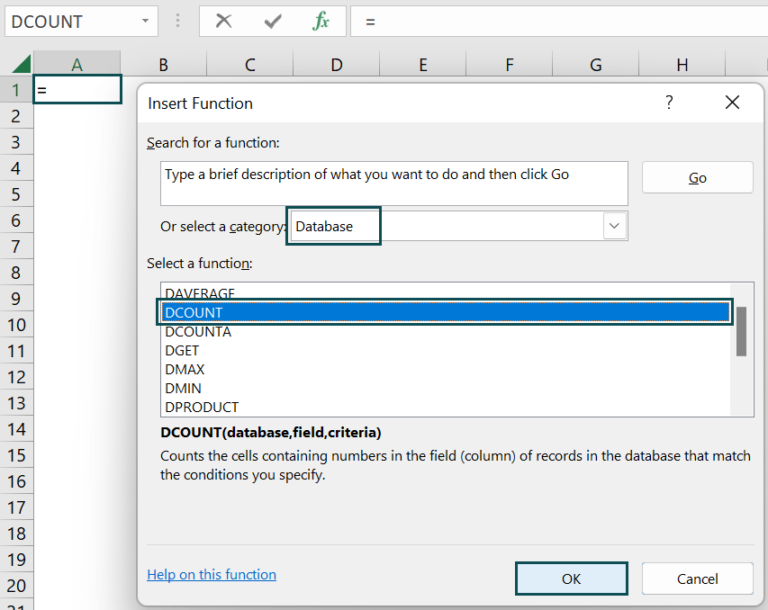# DCOUNT Excel FunctionArticle byExcelMojo Team## What Is DCOUNT Function In Excel?

The DCOUNT Excel function counts the cells containing numbers in a database that matches specified conditions/criteria. The DCOUNT function in Excel is an inbuilt “Database” function, so we can insert the formula from the “Function Library” or enter it directly in the worksheet.

For example, we will use the DCOUNT Function to calculate cells containing numerical values for the following values in column A.

Select cell B2, enter the formula =DCOUNT(A1:A3,A1,A2:A3), and press the “Enter” key.

The result is ‘2’, as shown above.

###### Key Takeaways
• The DCOUNT Excel function counts the numeric values selected from a database table-like array or range.
• The function can have multiple rows in the criteria argument.
• For the function to execute without errors, the “database” and “criteria” arguments in the header must be the same.
• The field value can be in double quotes.
• We can place the criteria data anywhere in the sheet, but it’s good to put them on the database.
• The “database” and “criteria” data should have at least one-row gap in between.

### DCOUNT() Excel Formula

The syntax of the DCOUNT Excel formula is,

The arguments of the DCOUNT Excel formula are,

• database: It is a mandatory argument. It is the range of cells that forms the list of data in the sheet.
• field: It is a mandatory argument. It is the selected column that is used in the calculation.
• criteria: It is a mandatory argument. It is the condition that is specified to calculate the function.

### How To Use DCOUNT Excel Function?

We can use the DCOUNT function in 2 ways, namely,

1. Access from the Excel ribbon.
2. Enter in the worksheet manually.

#### Method #1 – Access from the Excel ribbon

Choose an empty cell for the output → select the “Formulas” tab → click the “Insert Function” group, as shown below.

The “Insert Function” window appears. Now, choose the “Database” option from the “select a category:” drop-down.

Then, select the “DCOUNT” function from the “Select a function:” group → and click “OK”, as shown below.

The “Function Arguments” window appears. Enter the arguments in the “Database”, “field”, and “Criteria” fields → click “OK”, as shown below.

#### Method #2 – Enter in the worksheet manually

1. Select an empty cell for the output.
2. Type =DCOUNT( in the selected cell. [Alternatively, type =D or =DC and double-click the DCOUNT function from the list of suggestions shown by Excel.
3. Enter the arguments as cell values or cell references and close the brackets.
4. Press the “Enter” key.

Let us take an example to learn its application.

We will calculate the output by counting numeric values using DCOUNT Excel function for the values.

In the table, the data is,

• Column A contains the Value.
• Column B displays the Output.

The steps to calculate the value using the DCOUNT function are:

1. Select cell B2, and enter the formula =DCOUNT(A1:A6 i.e., the database value.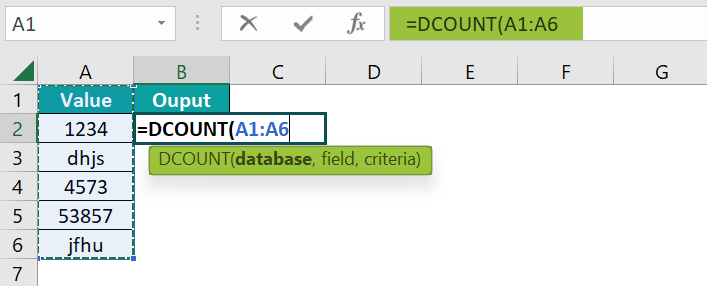2. Enter the value of ‘field’ as A1. The formula now is =DCOUNT(A1:A6,A1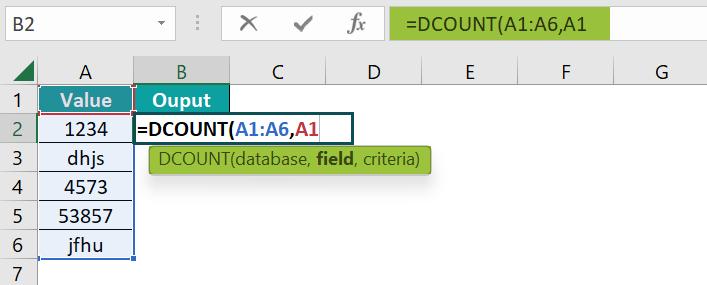3. Enter the value of ‘criteria’ as A2:A6, and close the brackets. The complete formula is =DCOUNT(A1:A6,A1,A2:A6).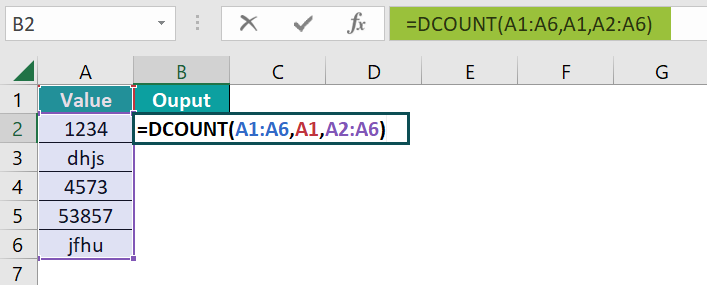4. Press the “Enter” key. The result is “3”, as shown below.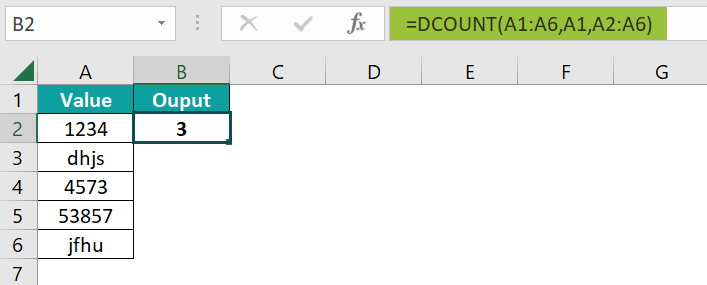[Output Observation: In cell B2, the criteria is to check for cells with numeric values in the Value field [A1]. Rows 2, 4, and 5 have numerical values, so the count is returned as 3.]

### Examples

We will understand some advanced scenarios using the DCOUNT Excel function examples.

#### Example #1

We will calculate the output by counting numeric values using the DCOUNT function for the values in different formats, such as strings, numbers, dates, time, and numbers in decimals and percentages.

In the table, the data is,

• Column A contains the Value.
• Column B displays the Output.

The procedure to calculate the value using the DCOUNT formula is,

Select cell B2, enter the formula =DCOUNT(A1:A10,A1,A2:A10), and press the “Enter” key.

[Note: The value of ‘database’ is A1:A10, ‘field’ is A1, and ‘criteria’ is A2:A10.]

The result is “6”, as shown above.

#### Example #2

We will calculate the output by counting numeric values using the DCOUNT function for the values as strings.

In the table, the data is,

• Column A contains the Value.
• Column B displays the Output.

The procedure to calculate the value using the DCOUNT formula is,

Select cell B2, enter the formula =DCOUNT(A1:A6,A1,A2:A6), and press the “Enter” key.

[Note: The value of ‘database’ is A1:A6, ‘field’ is A1, and ‘criteria’ is A2:A6.]

The result is “0”, as shown above. There is no numeric number in the cells, so the output is 0.

#### Example #3

We will calculate the output as the “#VALUE!” error using the DCOUNT function for the given values.

In the table, the data is,

• Column A contains the Value.
• Column B displays the Output.

The procedure to calculate the value using the DCOUNT formula is,

Select cell B2, enter the formula =DCOUNT(A1:A4,A1,), and press the “Enter” key.

[Note: The value of ‘database’ is A1:A4, and ‘field’ is A1.]

The result is a #VALUE!” error”, as shown above. If the criteria range is left blank, the #VALUE! Error occurs.

### Important Things To Note

• We get the “#VALUE!” error when,
• The criteria argument is blank.
• The cell range is incorrect.
• If one of the arguments is a text or in double-quotes.
• The DCOUNT() counts only the non-blank or numeric values.
• We get the “#Name?” error when the function name is incorrectly entered.

1. What does the DCOUNT Excel function mean?

The DCOUNT function counts the numeric values from the database that fulfills the set criteria. Like the COUNT function that returns the count of numeric values, the DCOUNT function also returns the count of values. However, the DCOUNT() checks for the criteria and then counts the cells that meet the criteria.

The syntax of the DCOUNT function is =DCOUNT(database,field,criteria)

2. How to insert the DCOUNT Excel function?

We can insert the DCOUNT function as follows:
1. Select an empty cell for the output.
2. Type =DCOUNT( in the selected cell. [Alternatively, type =D or =DC and double-click the DCOUNT function from the list of suggestions shown by Excel.
3. Enter the arguments as cell values or cell references and close the brackets.
4. Press the “Enter” key.

For example, we will calculate the output by counting numeric values using the DCOUNT function.

In the table, the data is,
Column A contains the Value.
Column B displays the Output.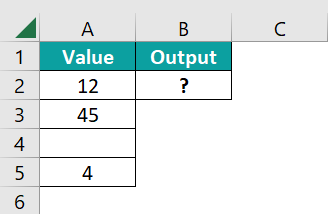The procedure to calculate the value using the DCOUNT formula is,
Select cell B2, enter the formula =DCOUNT(A1:A5,A1,A2:A5), and press the “Enter” key.
[Note: The value of ‘database’ is A1:A5, ‘field’ is A1, and ‘criteria’ is A2:A5.]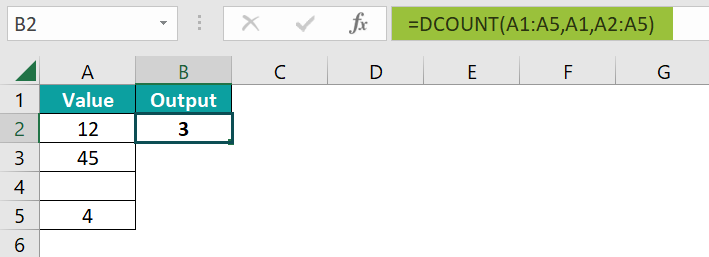The result is “3”, as shown above.

3. Where is the DCOUNT Excel function?

The DCOUNT function is found in the “Formulas” tab, as shown below.

Choose an empty cell for the output → select the “Formulas” tab → click the “Insert Function” group, as shown below.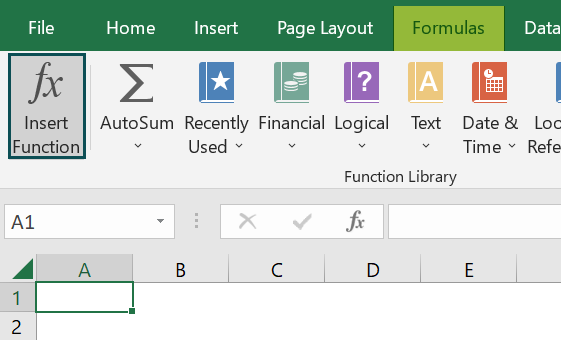The “Insert Function” window appears. Now, choose the “Database” option from the “select a category:” drop-down.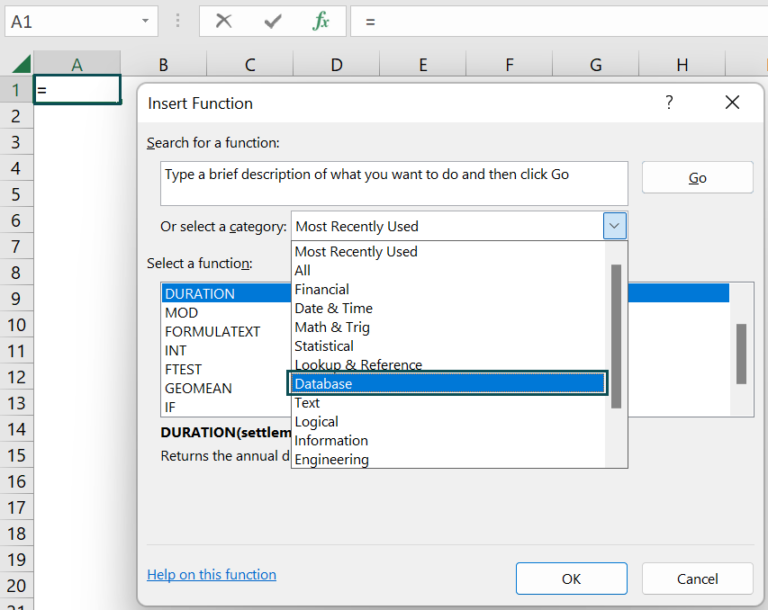Then, select the “DCOUNT” function from the “Select a function:” group → and click “OK”, as shown below.